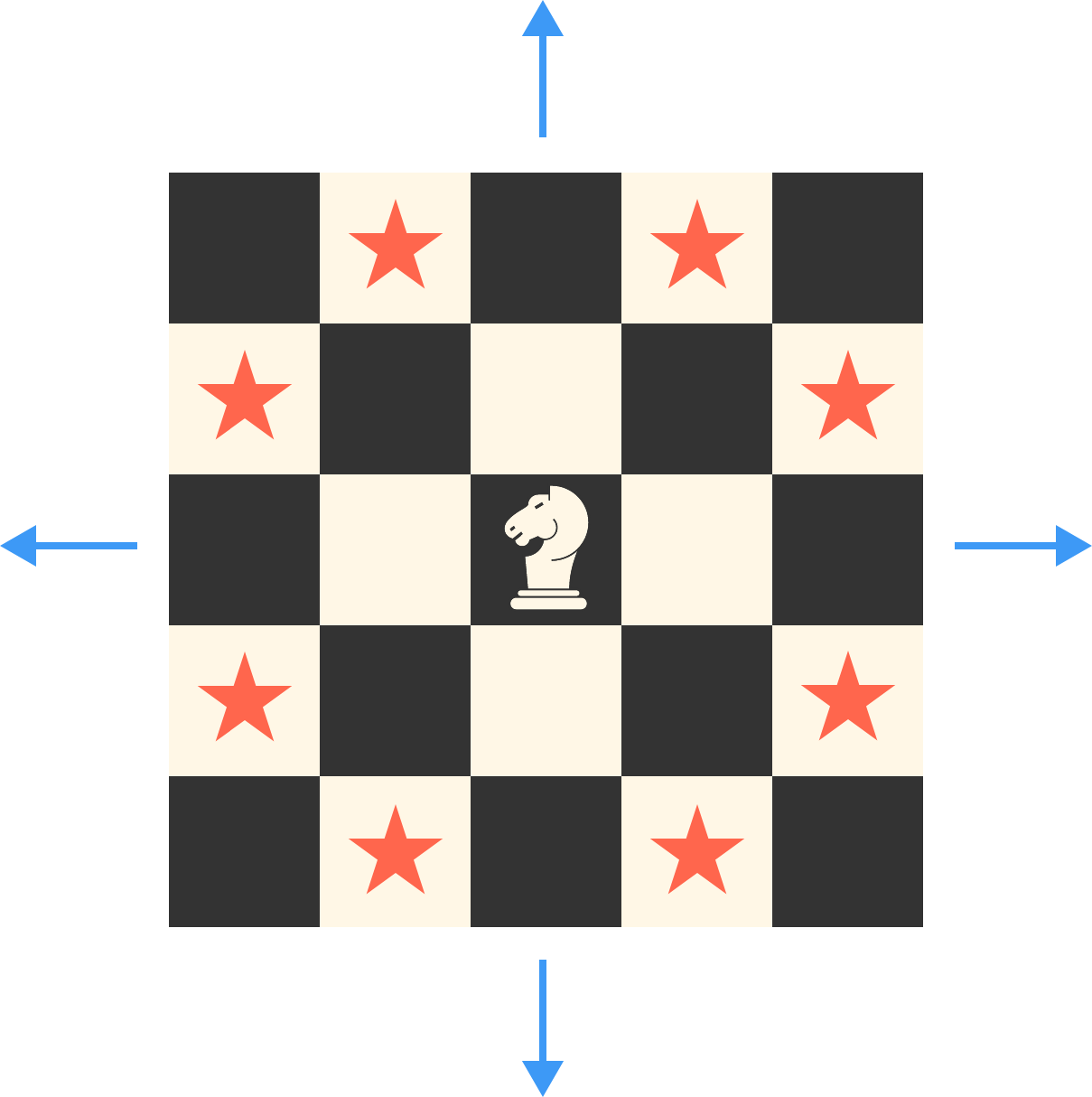Knights to Infinity

A knight is placed at the center of an infinitely large chess board. For large $n,$ the number of squares on which the knight can be after exactly $n$ moves is asymptotically $a \cdot n^b$ for real numbers $a$ and $b.$ Find $a+b.$Note: A chess knight moves in an "L" shape either 1 square vertically and 2 squares horizontally or 2 squares vertically and 1 square horizontally, as indicated by the stars above.

×